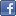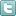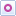EE Times-India > Power/Alternative Energy

Power/Alternative Energy# How to measure small LCs with an oscilloscope

Posted: 30 Mar 2016Print Version

Keywords:SMT inductor  LCR bridge  inductor  function generator  LC tank

In this case the capacitor in parallel with the inductor is 1nF and the resulting frequency is 156kHz. From the resonance equation f = 1 / [2π(LC)0.5], the inductor calculates to be 1mH. This technique can be used to measure inductance down to the nanohenry region—the known parallel capacitor might need to be increased accordingly to maintain a reasonable ring frequency within the scope's bandwidth.

Measuring unmarked capacitors is a bit easier technically, but physically more difficult. Assuming unmarked surface mount capacitors, they must first be carefully removed from the original PCB with a heat gun or a dual soldering iron. Their small size and fragility requires soldering fine-gauge wires to them, but a better fixture can be made by scoring single-sided copperclad with a Dremel cutting disc to form small pads to which the unknown capacitor and short test leads can be soldered.

A voltage step is applied to the capacitor using the following setup—again, the function generator can be replaced by a breadboarded logic edge generator; all we need is a voltage step with a risetime much faster than the resulting risetime of the measurement, and a period between edges long enough for the transient response to complete:This gives the classic voltage vs. time exponential charging curve. One RC time constant is at the point where the charging capacitor voltage reaches 63 per cent of the final voltage.Adjust the scope's vertical for a display that eventually rises to eight divisions. At this scale, the 63 per cent point falls conveniently near the fifth graticule division. In the above example, the cursors show that from start to 62.5 per cent is 300ns. Since the resistor is 10K, the capacitance C = t/R = 30 pF.

Now a probe correction must be applied by subtracting the known probe capacitance from the measured value to obtain the actual value of the capacitor under test. For a 15pF probe, the unknown capacitor must be 15pF.

The same capacitor measured with a higher quality 7.5pF probe produced the following curve:Subtracting the probe's 7.5pF from the measured 22.8pF gives 15.3pF for the unknown capacitor—close enough.

What if you don't know the probe capacitance? No problem—remove the capacitor under test and measure only the probe capacitance using the same method!

Note: Glen Chenier, the author, passed away on January 5, 2016.

Comment on "How to measure small LCs with an osc..."

Top Ranked Articles

WebinarsVisit Asia Webinars to learn about the latest in technology and get practical design tips.

Search EE Times India
Services

Go to topConnect on FacebookFollow us on TwitterFollow us on Orkut
﻿